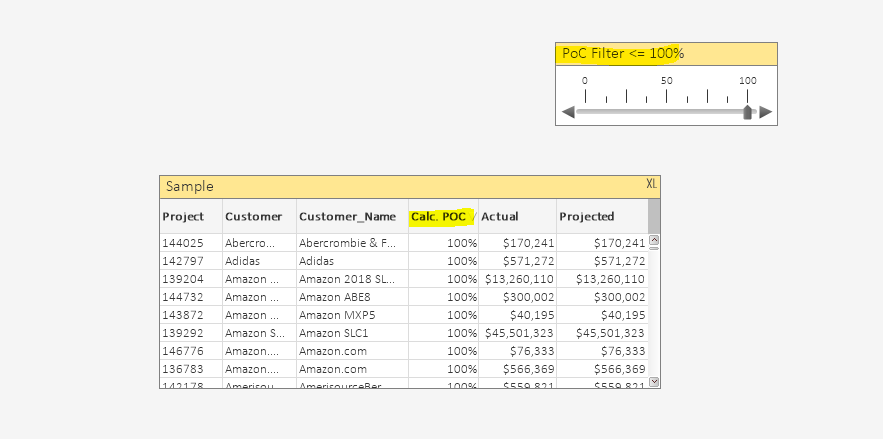# New to QlikView

Discussion board where members can get started with QlikView.

Announcements
Coming your way, the Qlik Data Revolution Virtual Summit. October 27-29. REGISTER
cancel
Showing results for
Did you mean:
HighlightedCreator III

## Slider and Straight table

Hello,

I've created a slider and straight table as shown below.Can anyone tell me how can I apply the changes so that the slider change will filter the table using "Calc. POC" column as highlighted above? Attached is the file for reference

thanks

Labels (2)

• ### straight table

2 Solutions

Accepted Solutions
HighlightedPartner

Simplest way
change project dimension to below

```=if(
Aggr((Sum({<[ORA DAY VERSION]={">=\$(vCurrentMonthStart)<=\$(vCurrentMonthEnd)"}>}[ORA Actual])
/
Sum({<[ORA DAY VERSION]={">=\$(vCurrentMonthStart)<=\$(vCurrentMonthEnd)"}>}[ORA Projected])
),[ORA Project Number],Engineering_Manager)*100<=var_PoC_Slider,
[ORA Project Number])```

not sure if thats the most efficient way.
Will check if theres a set analysis way to achieve it

HighlightedPartner

Take a look at the attached qvw...

I updated the [ORA Project Number] to be calculated based on Calc. POC expression; if less or equal than ... show the dim value otherwise make it null.

I hope you find it useful

4 Replies
HighlightedPartner

You can use set analysis to do so.

But exactly how depends on your data.

can you share a sample of your data and the expression used in your table

HighlightedCreator III

@dilipranjith  I've attached the file in the question.

HighlightedPartner

Simplest way
change project dimension to below

```=if(
Aggr((Sum({<[ORA DAY VERSION]={">=\$(vCurrentMonthStart)<=\$(vCurrentMonthEnd)"}>}[ORA Actual])
/
Sum({<[ORA DAY VERSION]={">=\$(vCurrentMonthStart)<=\$(vCurrentMonthEnd)"}>}[ORA Projected])
),[ORA Project Number],Engineering_Manager)*100<=var_PoC_Slider,
[ORA Project Number])```

not sure if thats the most efficient way.
Will check if theres a set analysis way to achieve it

HighlightedPartner

Take a look at the attached qvw...

I updated the [ORA Project Number] to be calculated based on Calc. POC expression; if less or equal than ... show the dim value otherwise make it null.

I hope you find it useful